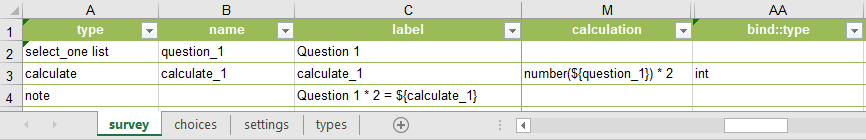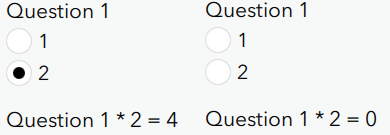# Directly Reference Select_One In Calculation

190
2
02-13-2020 05:57 PMbyEsri Contributor

Since the select_one question type does not support 'bind::type = int or decimal' at the moment and only returns a string value, currently a hidden or calculate question need to be added to convert the string to numeric values, if a following mathematical equation need to reference the select_one question.

There's a method to directly reference the select_one question in a mathematical equation, using the 'number()' function. The 'number()' function converts string value to numeric value, and moreover, it returns the value '0' when the question being referenced is unanswered. My current finding is, the question (select_one or calculate) being referenced has to be a string field type, otherwise the 'number()' function will return an empty value if the referenced question has not been answered.

This is confusing, if number('') = 0, why number(NaN) = NaN?

Edit: Perhaps NaN is treated as Number in XML, and number() does not convert numeric value. But that still doesn't explain why number('') = 0.

This method is very useful when we need to include answers of many non-required questions in a complex calculation, because the usual method (using a hidden question for conversion) not only creating many fields but also would return a NaN value (unanswered question) and fail the calculation - this can be solved by using a 'coalesce(\${question},0)' statement by the way.

My concern is, is there any pitfall or bug in this method and cause it not working? Especially when the select_one question is unanswered and returns an empty value.Any comment would be greatly appreciated. Ismael Chivite Jim Moore Ben Turrell

Kind Regards,

Sun

Tags (3)
2 RepliesbyEsri Regular Contributor

Hi Jing Sun‌, it looks like James Tedrick‌ has responded to a similar question on a separate post - just including the link to it here for the benefit of other visitors to this thread.

Cheers,

JimbyEsri Contributor

Hello Jim,

Thank you.

To summarise:

Number(\${question}) = 0  when \${question} is a string question & returns an empty string ('').

Number(\${question}) = NaN when \${question} is a integer/decimal question & returns an empty value (NaN). My research shows that an empty value (NaN) is treated as a numeric value, and number() does nothing to the numeric value, hence the output is NaN if the input is NaN.

Cheers,

Sun